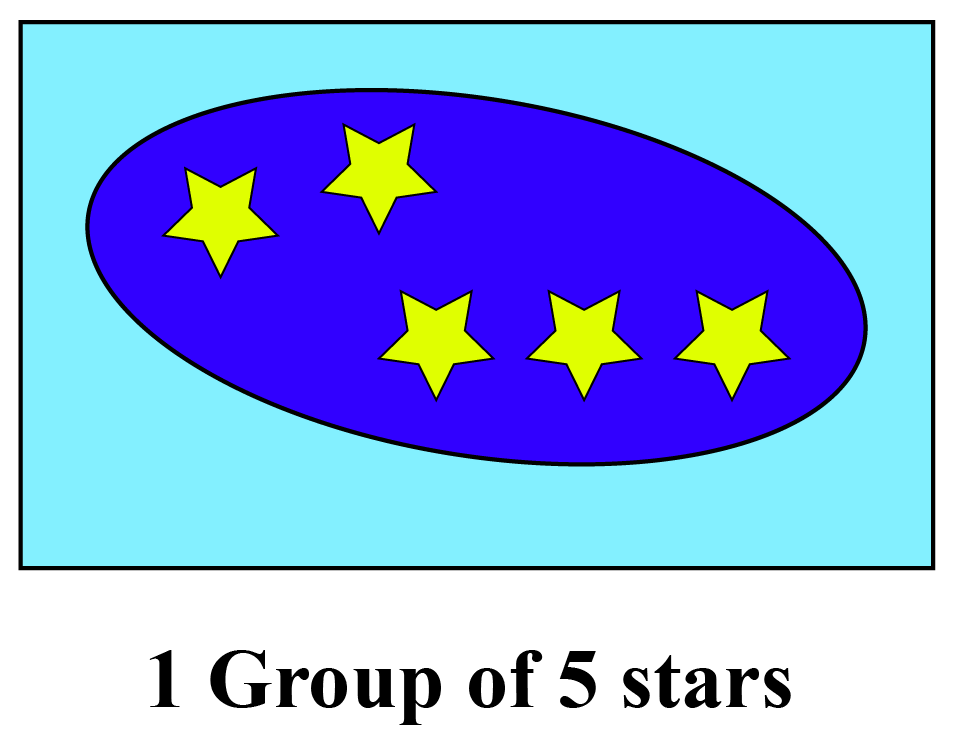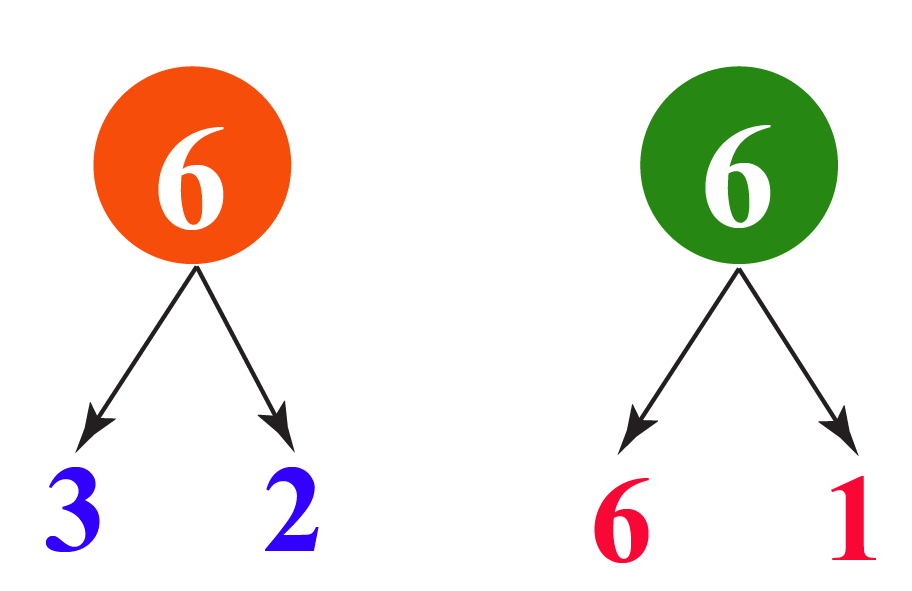# How to find out if a number is a prime number?

How to find out if a number is a prime number?
Go back to  'Prime Numbers'

In this lesson, we will learn about prime  and composite numbers, how to find if a number is prime or not, prime number definition, prime number examples, and interactive questions at the end.

Watch this video to know what prime numbers are,

## Lesson Plan

 1 How to Know if a Number is Prime or Not? 2 Important Notes 3 Tips and Tricks 4 Solved Examples 5 Interactive Questions

## How to Know if a Number Is Prime or Not?

A number that cannot be divided into equal groups is called a prime number. In other words, a number that is divisible only by 1 and itself is called a prime number.

So what do we call a number that is not prime?

A number that has factors other than one and itself is called a composite number.

### Definition

Any whole number that is divisible only by 1 and itself is called a prime number.

For example, 5 has only two factors: 1 and 5.We cannot divide 5 into equal groups.

5 can be factorized as:

\begin{align} 5 = 1\times5\end{align}

A composite number has many other factors other than 1 and itself.

For example, the number 6 has 1,2,3, and 6 as factors.6 can be factorized as

\begin{align} 6 = 1\times6\end{align}

\begin{align} 6 = 3\times2\end{align}

Since 6 has more than two factors (other than 1 and 6), it is a composite number.

### Examples of Prime Numbers

Some examples of prime numbers are 2,3,5,7,11,17.

### Is 1 a Prime Number or a Composite Number?

1 is neither a prime nor a composite number.

Since 1 has only 1 factor, it cannot be a prime number.

It is not composite because it does not have more than two factors.

## How to Determine if a Number is Prime or Not?

A prime number has exactly two factors. They are 1 and the number itself.

For example,7 and 17 have exactly two factors. Therefore, they are prime numbers.

Whereas, the number 6 has four factors (1,2,3,6)Therefore, 6 is a composite number.

### Twin Prime Numbers

Any two prime numbers that have one composite number in between them are called twin prime numbers.

For example,

(3,5), (5,7), (11,13) are twin prime numbers.

### Co-Prime Numbers

A pair of numbers that have no common factors other than 1 are called co-prime numbers. They can have both prime and composite numbers.

For example,

(5,9), (7,11), (18,33), (23,49)

More Important Topics
Numbers
Algebra
Geometry
Measurement
Money
Data
Trigonometry
Calculus